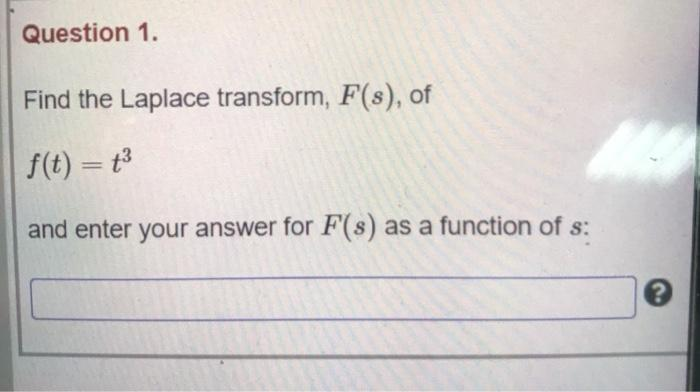### Create an Account

Already have account?

### Forgot Your Password ?

Home / Questions / Question 1. Find the Laplace transform, F(s), of f(t) = t3 and enter your answer for F(s) ...

# Question 1. Find the Laplace transform, F(s), of f(t) = t3 and enter your answer for F(s) as a function of s:

Question 1. Find the Laplace transform, F(s), of f(t) = t3 and enter your answer for F(s) as a function of s:Apr 15 2021 View more View Less

#### Answer (Solved)Subscribe To Get Solution